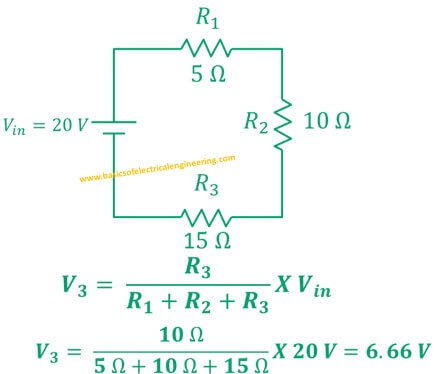# Basics of Electrical Engineering

Learn the basics of Electrical Engineering.

The electrical current always remains same in the series components. However, the voltage doesn't remain same in series components. The voltage divider rule is used to find the voltage divided across different components. Today you'll learn:
1. Statement of voltage divider
2. General formula of voltage divider rule
3. VDR for two resistors
4. VDR for three series resistors
5. Examples of voltage divider rule
6. FAQ's

### Voltage divider Statement and formula

The magnitude of divided voltage in a series circuit depends on the magnitude of resistance.

A series circuit is the one where one end of component connects to other and there is no other component in between them.

#### General formula

The general formula for calculating the voltage divider in a series circuit with 'n' resistors is:Let's simplify the general formula for two resistors.

The circuit below displays a circuit with two resistors R1 and R2. The source Vin powers circuit.We are interested to determine the voltage which is dropped across both resistors. Let's consider V1 is across dropped across R1 and V2 is across R2.
The formulas are:Let's solve an example to better understand it.

Example # 1: A circuit has two series resistors having their resistances 5 Ω and 10 Ω. Find the voltage across 10 Ω resistor when an 8 V source is connected to the input.
Solution:

In the similar fashion, we can apply the voltage divider rule to three, four, five or any number of resistors. The formula to calculate voltage  V1, V2, and V3 across R1, R2, and R3 respectively is:
Example # 2: Three resistors of 5, 10, 15 Ω are joined in series to 20 V source. Find the current across R3.
Solution:### Frequently asked questions

Question # 1: What is voltage divider equation?
Answer: The basic VDR formula: Vx = [Rx/Rt] *  Vin is referred to voltage divider equation.

Question # 2: How to apply voltage divider in parallel circuits?
Answer: Voltage always remains same in parallel. We don't need to apply VDR in parallel circuits.

Question # 3: What is loaded voltage divider?
Answer: A circuit in which some load is connected to one of the voltage divider resistors is referred as loaded voltage divider. You can use a loaded VDR calculator to solve this.

#### 5 comments:

1.HELPFUL ,GOOD!!

2.For parallel same method

3.Very Helpful

4.You are timely saver, I will stick to this site until I graduate

5.Good finally I get best site